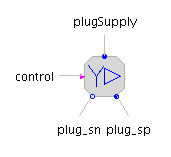Hydraulic Fluid Properties - MapleSim Help

Hydraulic Fluid Properties

Generic fluid properties reference model for the hydraulic systemDescription The Hydraulic Fluid Properties component defines the properties of a hydraulic fluid as well as global settings for the hydraulic system. In the model workspace, place this component at the same level as the hydraulic components or subsystem to which you want to assign the hydraulic fluid properties. For example, if you place this component at the top level of the model, the hydraulic fluid properties will apply to all of the hydraulic components and subsystems in your model. If you place this component within a subsystem, the hydraulic fluid properties defined in the component will apply to the hydraulic components in that subsystem only and all nested subsystems.Fluid Parameters

 Name Default Units Description Modelica ID $\mathrm{\rho }$ $850$ $\frac{\mathrm{kg}}{{m}^{3}}$ Density rhoFluid $K$ $8·{10}^{8}$ $\mathrm{Pa}$ Bulk modulus ElFluid $\mathrm{\nu }$ $1.8·{10}^{-5}$ $\frac{{m}^{2}}{s}$ Kinematic viscosity nuFluidGlobal Settings

The global settings ${p}_{\mathrm{atm}}$ and ${A}_{\mathrm{min}}$ are used by some components to set the value of the atmospheric pressure and the minimum allowable orifice area.  As with the fluid parameters, these settings apply to all hydraulic components at the same or lower level in a subsystem hierarchy.

 Name Default Units Description Modelica ID ${p}_{\mathrm{atm}}$ $101325$ $\mathrm{Pa}$ Atmospheric pressure patm ${A}_{\mathrm{min}}$ $1·{10}^{-12}$ ${m}^{2}$ Minimum orifice area AminSummary Variables

These parameters set the units used by the internal summary variables. See Hydraulic Summary Variables.

 Name Default Description Modelica ID Summary pressure unit $\mathrm{kPa}$ Unit for internal pressure pressUnitSummary Summary flow rate unit $\frac{\mathrm{liter}}{s}$ Unit for internal flow rate flowUnitSummary